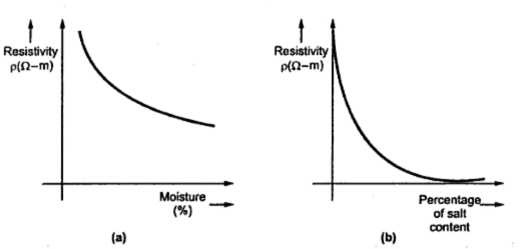### Resistance of Grounding Systems

The ground resistance of an electrode system is nothing but the resistance between the system of electrodes and other infinitely large electrode in the ground at infinite spacing. The ground resistance is also decided by soil resistivity which is electro-physical property. The soil resistivity depends on various factors such as type of soil, moisture content, dissolved salt etc. It is also affected by grain size and its distribution. The temperature and pressure are the other factors which have influence on soil resistivity.
In practice it is not possible to have homogeneous soil. The apparent resistivity is hence defined for an equivalent homogeneous soil.
The moisture content in the soil is also a variable factor which changes with sensors. With increase in moisture content, resistivity of soil reduces. To overcome this difficulty, the grounding systems may be installed near to the permanent water level so that the change in resistivity due to change in seasons is minimized.
With decrease in temperature, the soil resistivity increases and the discontinuity is observed at freezing point. The quantity of salt dissolved in the moisture reduces the resistivity appreciably. Various salts have different effects on the soil resistivity. With finer graining, the resistivity of soil reduces while the large value of pressure decreases soil resistivity.
The variation of soil resistivity with moisture and salt content is shown in the Fig. 1(a) and (b).Fig 1 Variation of soil resistivity with moisture and salt content
Resistance of Grounding point Electrode
Consider a hemispherical electrode buried in the soil as shown in the Fig. 2(a) which is simplest possible from of electrode.Fig 2
The total resistance in this case can be divided into three parts which are viz.
i) resistance of conductor ii) contact resistance between the surface of electrode and main body of earth which is negligible  iii) resistance of body of earth surrounding the electrode which is the main part.
Let I be the current dissipated by the electrode. This current spreads out radically in the earth. At a distance x from the centre of hemispherical the current density is given by,
The electric field strength e due to current density i is given by,
The line integral of the field strength e from the surface of sphere of radius R to the distance x gives the voltage V.
The variation of e, V and i with respect to distance x is shown in the Fig. 2(b).
The voltage between the hemispherical electrode and a point at infinity i.e. x = ∞ is given by,
The earth resistance is therefore given by,
1.1 Generalised Equation
Consider a system with respect two electrodes having their potentials as V1 and V2. Let V be the potential at any point in the medium having resistivity as ρ. Let ψ be electrostatic potential.
Total flow of current from the electrode in the outward direction is,
Here ds is an element of the electrode surface.
If Q is the charge on this electrode then by Gauss theorem.
The total flow of current is given by,
If C is the capacitance between the electrodes in air then
R is the resistance between the electrodes which is given by,

Suppose C is the capacitance of the single electrode with the return electrode at infinity then R is the resistance of earth for single electrode.
If we consider a sphere of radius S then the capacitance of sphere in air is equal to radius S.
R = ρ/4πS
If this electrode is hemispherical and buried with its lower half in the earth then the resistance is given by,
R = ρ/2πS
In general the equation for any electrode is given by,
Where C is the electrostatic capacity of the electrode along with its image above the surface of the earth. The combined electrode is considered as in air.